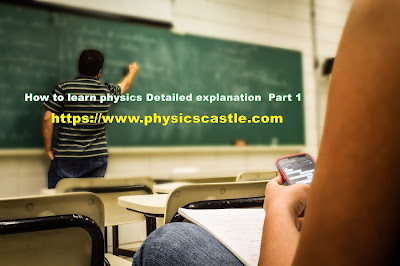# How to learn physics Detailed explanation : Part 1

## How to learn physics Detailed explanationhttps://www.physicscastle.com

### Simplify how to learn physics:

It’s great that you want to learn: how to learn physics - it’s one of the most intellectually stimulating subjects around, The best and only way to study physics is to do physics problems, Solving the problems forces you to know the formulas and understand the math, It creates the landscape in which you will learn the concepts, develop your intuitive sense of how physics works, and fathom the rich interconnection of the ideas.

If you read the textbook, or listen to a lecture, you get an idea of what the landscape looks like, but it is not the experience of physics, It is like the difference between watching a TV program about the Grand Canyon, and actually walking around in it.

I’ve tried a lot of ways to learn about physics throughout the past four years. As a sophomore, in a high school with a less than stellar science department, I became interested in physics. I started feeding that interest by reading basic online articles and by watching some online videos.

Slowly the material there became repetitive at which point I subscribed to Physics Today,I would read the monthly issues with excitement, but I rarely understood anything below the surface level.

### The best way to learn about physics:

Finally, I went off to college and enrolled in an introductory classical mechanics course. That is where everything started to click. After struggling (quite a bit) through the beginning of the semester, I have completed the two introductory courses in classical mechanics and electromagnetism, and I will be starting thermodynamics and special relativity in the fall.

What I’m trying to say is that the best way to learn about physics is to go straight to the basics. Trying to read about quantum mechanics and general relativity may seem like a really cool and exotic way to satiate your interest, but it won’t truly make sense until you have the basics under your belt. Whether you take classes at a college or you simply pick up a book on mechanics that is the best place to start.

In the words of Richard Feynman,) you are unlikely to discover something new without a lot of practice on old stuff (.

### Relationship between learning physics and mathematics:

The difference is small, especially at the introductory level. But as you get further in physics, the difference becomes more important, and sometimes a problem is very hard if you treat it as a physics-is-math problem, but very easy if you treat it as a physics problem.

#### What do I mean?

For sure, you see a lot of math in physics. Take the concept of momentum, for example:(p=mv) , where p is an object’s momentum, m is the object’s mass, and v is the object’s velocity, If you think physics is math, you’ll just kind of add that formula to your inventory of other formulas. When a problem asks something about momentum, you’ll dial up your inventory of momentum-related formulas, and make mathematical manipulations until you get what the problem is asking for.

But you’re not understanding that formula in terms of physics, Don’t get me wrong, you should have an inventory of formulas, and when you solve a problem you should make mathematical manipulations with those formulas to get an answer. But having a little physical insight will help you find the right formulas and the right manipulations quicker.

So what else should you do? For example, you should explore the extreme ends of both variables, For example in the context of momentum, you should realize that a low-mass, high-velocity thing (like a bullet) can have the same momentum as a high-mass, low-velocity thing (like a slowly moving train). You should try to get a feel for what that means. Imagine what it feels like to try to stop a slowly moving train, imagine what it feels like to stop a quickly moving bullet.

These types of exercises help you gain physical intuition, so you know which concept to apply. For example, concepts which might be related to momentum include kinetic energy, force, impulse, and work. They’re all formulas involving mass and aspects of the object’s motion, for example. So if you just think of physics as mathematics, you might kind of lump all these formulas in a similar “inventory category” of formulas involving mass and motion.

Thus, when you’re handed a problem asking how fast an object is going given certain initial conditions, the physics-as-math type of person might blindly search through their inventory of formulas - a possibly long inventory- looking for a string of formulas with the right inputs that match the given information and the right output that provides the answer. But with physical insight, you’ll know instantly that such-and-such problem is a conservation of momentum problem, while so-and-so problem is a work/energy problem. In other words, your search space will be much smaller.

>> Top 10 books in physics pdf  💞💞👈